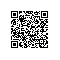# 三步教你搭建给黑白照片上色的神经网络 !（附代码）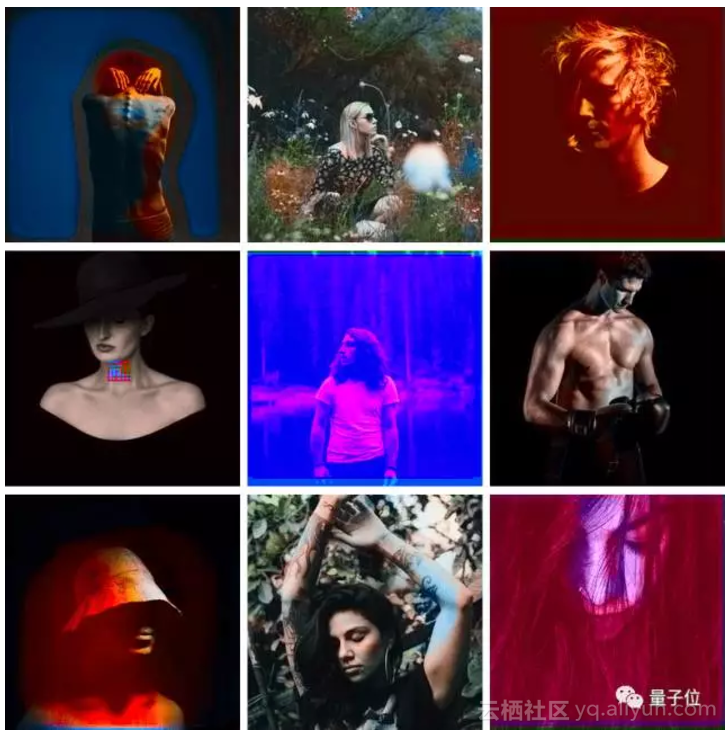FloydHub是个YC孵化的创业公司，号称要做深度学习领域的Heroku。它在GPU系统上预装了TensorFlow和很多其他的机器学习工具，用户可以按时长租用，训练自己的机器学习模型。免费版支持1个项目、每月20小时GPU时长、10G存储空间，用上色项目练个手足够了。

Alpha版本机器人的Jupyter Notebook代码、上述三个版本实现代码的FloydHub和GitHub地址，以及在FloydHub的GPU云上运行的所有实验代码，都在文末。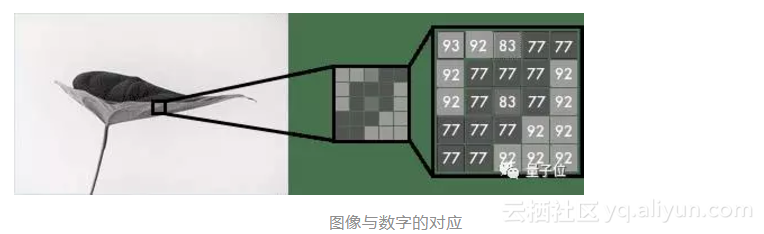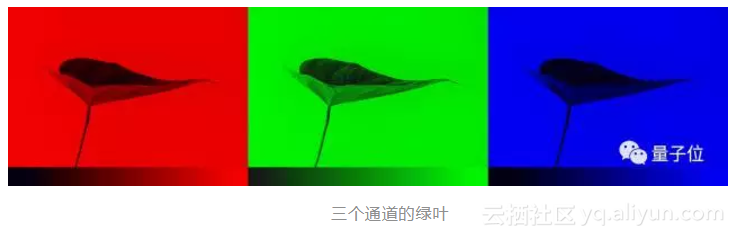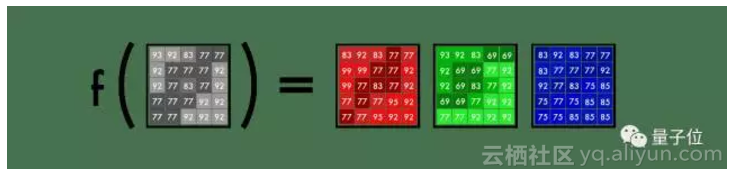Alpha版本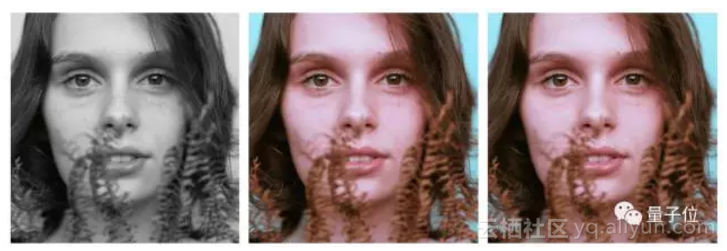1、颜色空间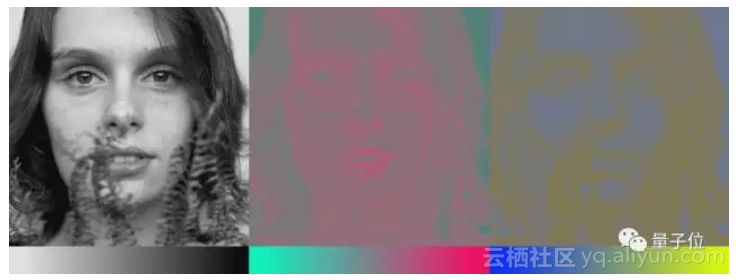2、从黑白到彩色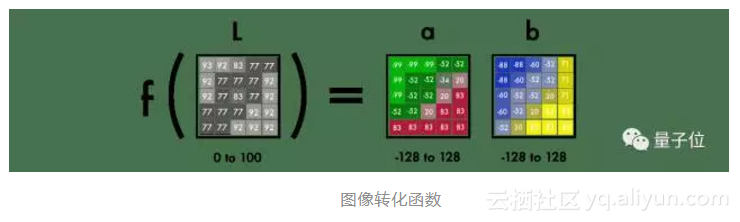3、在FloydHub上部署代码


4、Alpha版本

git clone https://github.com/emilwallner/Coloring-greyscale-images-in-Keras


cd Coloring-greyscale-images-in-Keras/floydhub
floyd init colornet


FloydHub Web控制台将在浏览器中打开，系统会提示你创建一个名为colornet的FloydHub新项目。完成后，返回终端并执行相同的初始化命令。

floyd init colornet


floyd run --data emilwallner/datasets/colornet/2:data --mode jupyter --tensorboard


• 我们在FloydHub的数据集目录（—data emilwallner / datasets / colornet / 2：data）中载入了一个公开数据集，你在FloydHub上查看并使用此数据集和许多其他公开数据集；
• 启用了Tensorboard (—tensorboard)；
• 在Jupyter Notebook下运行代码 (—mode jupyter)；
• 如果能使用GPU，你还可以将GPU (—gpu)添加到命令中，这样运行速度能提高50倍。

model.fit(x=X, y=Y, batch_size=1, epochs=1)


# Get images
image = np.array(image, dtype=float)

# Import map images into the lab colorspace
X = rgb2lab(1.0/255*image)[:,:,0]
Y = rgb2lab(1.0/255*image)[:,:,1:]
Y = Y / 128
X = X.reshape(1, 400, 400, 1)
Y = Y.reshape(1, 400, 400, 2)

model = Sequential()

# Building the neural network
model = Sequential()

# Finish model
model.compile(optimizer='rmsprop',loss='mse')

#Train the neural network
model.fit(x=X, y=Y, batch_size=1, epochs=3000)
print(model.evaluate(X, Y, batch_size=1))

# Output colorizations
output = model.predict(X)
output = output * 128
canvas = np.zeros((400, 400, 3))
canvas[:,:,0] = X[:,:,0]
canvas[:,:,1:] = output
imsave("img_result.png", lab2rgb(cur))
imsave("img_gray_scale.png", rgb2gray(lab2rgb(cur)))


floyd run --data emilwallner/datasets/colornet/2:data --mode jupyter --tensorboard


5、技术说明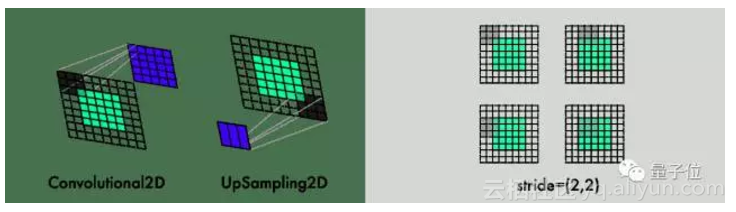for filename in os.listdir('/Color_300/Train/'):


datagen = ImageDataGenerator(
shear_range=0.2,
zoom_range=0.2,
rotation_range=20,
horizontal_flip=True)


batch_size = 50
def image_a_b_gen(batch_size):
for batch in datagen.flow(Xtrain, batch_size=batch_size):
lab_batch = rgb2lab(batch)
X_batch = lab_batch[:,:,:,0]
Y_batch = lab_batch[:,:,:,1:] / 128
yield (X_batch.reshape(X_batch.shape+(1,)), Y_batch)


model.fit_generator(image_a_b_gen(batch_size), steps_per_epoch=1, epochs=1000)


6、训练心得

• 先进行多次小批次实验，再尝试大批次实验。即使经过20-30次实验，我仍然发现很多错误。程序能运行并不意味着它能工作。神经网络中的问题通常比传统编程遇到的Bug更为麻烦。
• 多样化的数据集会使着色效果呈现棕色。如果数据集中图像比较相似，不需要设计很复杂的架构，就可以得到一个良好效果，但是其泛化能力十分糟糕。
• 重视图像形状的统一性。每张图像的分辨率必须是准确的，且在整个网络中保持成比例。开始时，所使用的图像分辨率为300，将它减半三次后分别得到150、75和35.5。因此，就丢失了半个像素，这导致了很多问题，后来才意识到应该使用4、8、16、32、64、256等这种能被2整除的数。
• 创建数据集。请禁用.DS_Store文件，不然会产生很多麻烦；大胆创新，最后我用Chrome控制台脚本和扩展程序来下载文件；备份原始文件并构建清理脚本。

Inception resnet v2是目前最强大的分类器之一，使用了120万张图像来训练该网络。我们提取了它的分类层，并将其与编码器的输出进行合并。因此，输入数据传给编码器的同时，也并行传输到resnet v2网络的分类层中。更多信息，请参考原论文：https://github.com/baldassarreFe/deep-koalarization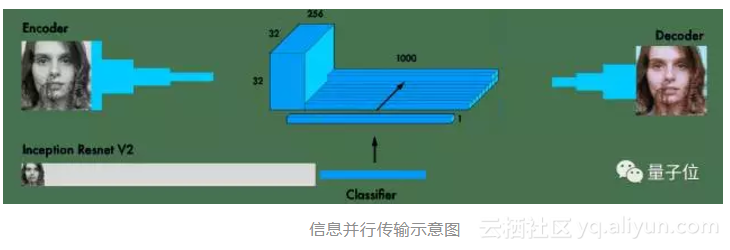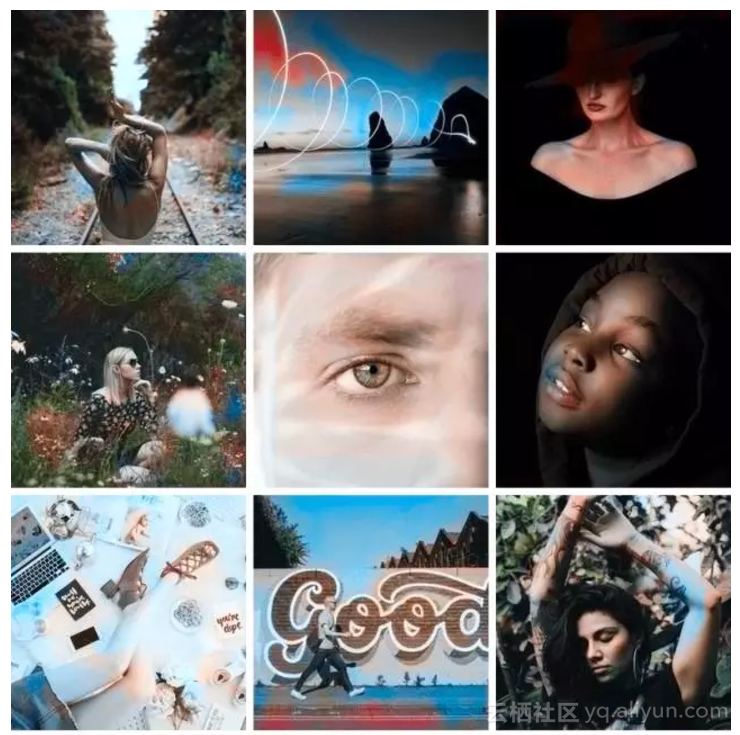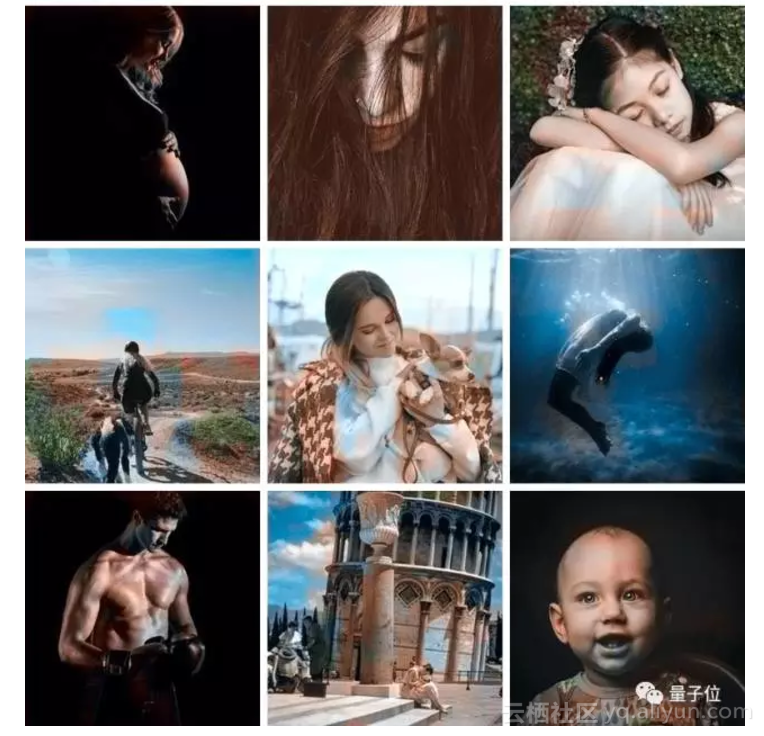# Get images
X = []
for filename in os.listdir('/data/images/Train/'):
X = np.array(X, dtype=float)
Xtrain = 1.0/255*X

inception = InceptionResNetV2(weights=None, include_top=True)
inception.graph = tf.get_default_graph()

embed_input = Input(shape=(1000,))

#Encoder
encoder_input = Input(shape=(256, 256, 1,))
encoder_output = Conv2D(64, (3,3), activation='relu', padding='same', strides=2)(encoder_input)
encoder_output = Conv2D(128, (3,3), activation='relu', padding='same')(encoder_output)
encoder_output = Conv2D(128, (3,3), activation='relu', padding='same', strides=2)(encoder_output)
encoder_output = Conv2D(256, (3,3), activation='relu', padding='same')(encoder_output)
encoder_output = Conv2D(256, (3,3), activation='relu', padding='same', strides=2)(encoder_output)
encoder_output = Conv2D(512, (3,3), activation='relu', padding='same')(encoder_output)
encoder_output = Conv2D(512, (3,3), activation='relu', padding='same')(encoder_output)
encoder_output = Conv2D(256, (3,3), activation='relu', padding='same')(encoder_output)

#Fusion
fusion_output = RepeatVector(32 * 32)(embed_input)
fusion_output = Reshape(([32, 32, 1000]))(fusion_output)
fusion_output = concatenate([encoder_output, fusion_output], axis=3)
fusion_output = Conv2D(256, (1, 1), activation='relu', padding='same')(fusion_output)

#Decoder
decoder_output = Conv2D(128, (3,3), activation='relu', padding='same')(fusion_output)
decoder_output = UpSampling2D((2, 2))(decoder_output)
decoder_output = Conv2D(64, (3,3), activation='relu', padding='same')(decoder_output)
decoder_output = UpSampling2D((2, 2))(decoder_output)
decoder_output = Conv2D(32, (3,3), activation='relu', padding='same')(decoder_output)
decoder_output = Conv2D(16, (3,3), activation='relu', padding='same')(decoder_output)
decoder_output = Conv2D(2, (3, 3), activation='tanh', padding='same')(decoder_output)
decoder_output = UpSampling2D((2, 2))(decoder_output)

model = Model(inputs=[encoder_input, embed_input], outputs=decoder_output)

#Create embedding
def create_inception_embedding(grayscaled_rgb):
grayscaled_rgb_resized = []
for i in grayscaled_rgb:
i = resize(i, (299, 299, 3), mode='constant')
grayscaled_rgb_resized.append(i)
grayscaled_rgb_resized = np.array(grayscaled_rgb_resized)
grayscaled_rgb_resized = preprocess_input(grayscaled_rgb_resized)
with inception.graph.as_default():
embed = inception.predict(grayscaled_rgb_resized)
return embed

# Image transformer
datagen = ImageDataGenerator(
shear_range=0.4,
zoom_range=0.4,
rotation_range=40,
horizontal_flip=True)

#Generate training data
batch_size = 20

def image_a_b_gen(batch_size):
for batch in datagen.flow(Xtrain, batch_size=batch_size):
grayscaled_rgb = gray2rgb(rgb2gray(batch))
embed = create_inception_embedding(grayscaled_rgb)
lab_batch = rgb2lab(batch)
X_batch = lab_batch[:,:,:,0]
X_batch = X_batch.reshape(X_batch.shape+(1,))
Y_batch = lab_batch[:,:,:,1:] / 128
yield ([X_batch, create_inception_embedding(grayscaled_rgb)], Y_batch)

#Train model
tensorboard = TensorBoard(log_dir="/output")
model.fit_generator(image_a_b_gen(batch_size), callbacks=[tensorboard], epochs=1000, steps_per_epoch=20)

#Make a prediction on the unseen images
color_me = []
for filename in os.listdir('../Test/'):
color_me = np.array(color_me, dtype=float)
color_me = 1.0/255*color_me
color_me = gray2rgb(rgb2gray(color_me))
color_me_embed = create_inception_embedding(color_me)
color_me = rgb2lab(color_me)[:,:,:,0]
color_me = color_me.reshape(color_me.shape+(1,))

# Test model
output = model.predict([color_me, color_me_embed])
output = output * 128

# Output colorizations
for i in range(len(output)):
cur = np.zeros((256, 256, 3))
cur[:,:,0] = color_me[i][:,:,0]
cur[:,:,1:] = output[i]
imsave("result/img_"+str(i)+".png", lab2rgb(cur))


floyd run --data emilwallner/datasets/colornet/2:data --mode jupyter --tensorboard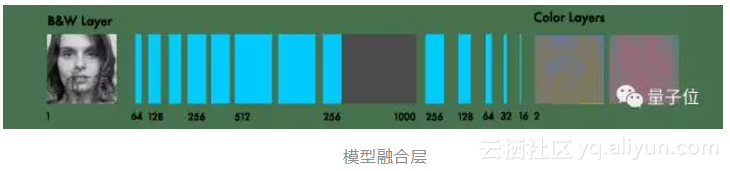inception = InceptionResNetV2(weights=None, include_top=True)
inception.graph = tf.get_default_graph()


grayscaled_rgb = gray2rgb(rgb2gray(batch))
embed = create_inception_embedding(grayscaled_rgb)


def create_inception_embedding(grayscaled_rgb):
grayscaled_rgb_resized = []
for i in grayscaled_rgb:
i = resize(i, (299, 299, 3), mode='constant')
grayscaled_rgb_resized.append(i)
grayscaled_rgb_resized = np.array(grayscaled_rgb_resized)
grayscaled_rgb_resized = preprocess_input(grayscaled_rgb_resized)
with inception.graph.as_default():
embed = inception.predict(grayscaled_rgb_resized)
return embed


yield ([X_batch, create_inception_embedding(grayscaled_rgb)], Y_batch)


model = Model(inputs=[encoder_input, embed_input], outputs=decoder_output)
e
ncoder_input会输入到编码器模型中；接着，编码器模型的输出会与融合层中的embed_input相融合；然后，这个融合输出会作为解码器模型的输入；最终，解码器模型会输出预测结果decode_output。

fusion_output = RepeatVector(32 * 32)(embed_input)
fusion_output = Reshape(([32, 32, 1000]))(fusion_output)
fusion_output = concatenate([fusion_output, encoder_output], axis=3)
fusion_output = Conv2D(256, (1, 1), activation='relu')(fusion_output)

在融合层中，首先将1000类输出层乘以1024（即32×32），这样就从inception模型的最后一层得到了1024行数据；接着，把2D重构成3D得到一个具有1000个类别对象的32x32网格；然后，将它与编码器模型的输出相连；最后，应用一个带有254个1X1内核的卷积网络，得到了融合层的最终输出。
<span style='display:block;text-align:center'>
**一些思考**
</span>
1. 不要逃避难懂的术语。我花了三天时间去搜索在Keras该如何实现“融合层”。因为这听起来很复杂，我不想面对这个问题，而是试图找到现成代码。
2. 多在网上请教他人。在Keras论坛中，我提出的问题没人回答，同时Stack Overflow删除了我的提问。但是，通过分解成小问题去请教他人，这迫使我进一步理解问题，并更快解决问题。
3. 多发邮件请教。虽然论坛可能没人回应，人们关心你能否直接与他们联系。在Skype上与你不认识的研究人员一起探讨问题，这听起来很有趣。
4. 在解决“融合层”问题之前，我决定构建出所有组件。以下是分解融合层的几个实验（https://www.floydhub.com/emilwallner/projects/color/24/code/Experiments/transfer-learning-examples）。

<span style='display:block;text-align:center'>
**下一步计划**
</span>

1. 微调另一个预训练好的模型；
2. 使用不同的数据集；
3. 添加更多图片来提高网络的正确率；
4. 在RGB颜色空间中构建一个放大器。构建一个与着色网络类似的模型，将深色调的着色图像作为输入，它能微调颜色以输出合适的着色图像；
5. 进行加权分类；
6. 应用一个分类神经网络作为损失函数。网络中错误分类的图片有一个相应误差，探究每个像素对该误差的贡献度。
7. 应用到视频中。不要太担心着色效果，而是要关注如何使图像切换保持协调。你也可以通过平铺多张小图像来处理大型图像。

1. 对于Alpha版本，只需将你的图片分辨率调成400x400像素，把名称改为woman.jpg，并替换原有文件。
2. 对于Beta版本和最终版本，在你运行FloydHub命令之前，要将你的图片放入Test文件夹。当Notebook运行时，你也可以通过命令行直接上传到Test文件夹。要注意，这些图像的分辨率必须是256x256像素。此外，你也可以上传彩色测试图像集，因为这个网络会自动把它们转换为黑白图像。

**相关链接**

1．Alpha版本机器人的Jupyter Notebook代码：
https://www.floydhub.com/emilwallner/projects/color/43/code/Alpha-version/alpha_version.ipynb

2．三个版本实现代码 - Floydhub传送门：
https://www.floydhub.com/emilwallner/projects/color/43/code

3．三个版本实现代码 - Github传送门：
https://github.com/emilwallner/Coloring-greyscale-images-in-Keras